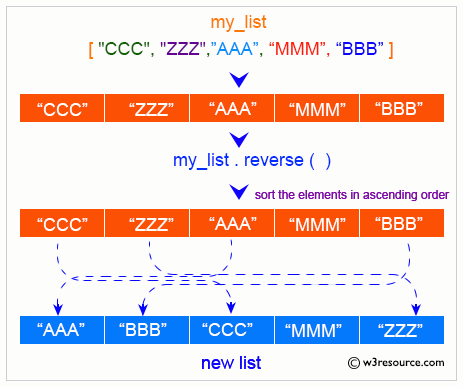# Python List: sort() Method

## sort() Method

The sort() method is used to sort the items of a given list.

Visual Explanation:Syntax:

`list.sort(*, key=None, reverse=False)`

Parameter:

• Key: Optional. A function that specifies the sorting criteria.
• reverse - Default value of reverse is False. False sort the list in ascending order and True sort the list in descending order.

Return Value:

The sort() method doesn't return any value. Rather, it modifies the original list.

Example 1: Sort a list in ascending/descending order

``````
colors = ['Red', 'Green', 'Orange', 'Pink']
print("Original List:")
print(colors)
colors.sort()
print('\nSort the said list in ascending order:')
print(colors)
print('\nSort the said list in descending order:')
colors.sort(reverse=True)
print(colors)
``````

Output:

```Original List:
['Red', 'Green', 'Orange', 'Pink']

Sort the said list in ascending order:
['Green', 'Orange', 'Pink', 'Red']

Sort the said list in descending order:
['Red', 'Pink', 'Orange', 'Green']
```

Example 3: Sort the list using key

``````
def test(data):
return data['Math']
students = [
{'V': 'Jenny Vang', 'Math': 99},
{'V': 'Allan Barker', 'Math': 100},
{'V': 'Ellie Ross', 'Math': 98},
{'V': 'Antoine Booker', 'Math': 99}
]
print("Original students list:")
print(students)
students.sort(key=test)
print("\nStudents list after sorting on Math marks:")
print(students)
``````

Output:

```Original students list:
[{'V': 'Jenny Vang', 'Math': 99}, {'V': 'Allan Barker', 'Math': 100}, {'V': 'Bradley Whitney', 'Math': 97}, {'V': 'Ellie Ross', 'Math': 98}, {'V': 'Antoine Booker', 'Math': 99}]

Students list after sorting on Math marks:
[{'V': 'Bradley Whitney', 'Math': 97}, {'V': 'Ellie Ross', 'Math': 98}, {'V': 'Jenny Vang', 'Math': 99}, {'V': 'Antoine Booker', 'Math': 99}, {'V': 'Allan Barker', 'Math': 100}]
```

Python Code Editor:

Previous: Python List reverse() Method
Next: Python List

Test your Python skills with w3resource's quiz

﻿

## Python: Tips of the Day

Kwargs:

**kwargs and *args are function arguments that can be very useful.

They are quite underused and often under-understood as well.

Let's try to explain what kwargs are and how to use them.

• While *args are used to pass arguments at an unknown amount to functions, **kwargs are used to do the same but with named arguments.
• So, if *args is a list being passed as an argument, you can think of **kwargs as a dictionary that's being passed as an argument to a function.
• You can use arguments as you wish as long as you follow the correct order which is: arg1, arg2, *args, **kwargs. It's okay to use only one of those but you can't mix the order, for instance, you can't have: function(**kwargs, arg1), that'd be a major faux pas in Python.
• Another example: You can do function(*args,**kwargs) since it follows the correct order.
• Here is an example. Let's say satelites are given with their names and weight in tons in dictionary format. Code prints their weight as kilograms along with their names.
```def payloads(**kwargs):
for key, value in kwargs.items():
print( key+" |||", float(value)*100)
payloads(NavSat1 = '2.5', BaysatG2 = '4')
```

Output:

```NavSat1 ||| 250.0
BaysatG2 ||| 400.0```

Since the function above would work for any number of dictionary keys, **kwargs makes perfect sense rather than passing arguments with a fixed amount.

```def payloads(**kwargs):
for key, value in kwargs.items():
print( key+" |||", float(value)*100)

sats={"Tx211":"3", "V1":"0.50"}
```Tx211 ||| 300.0